﻿ Polyglot data science
module Array

from Microsoft.FSharp.Collections
val filter : predicate:('T -> bool) -> array:'T [] -> 'T []

Full name: Microsoft.FSharp.Collections.Array.filter
val stock : obj
val map : mapping:('T -> 'U) -> array:'T [] -> 'U []

Full name: Microsoft.FSharp.Collections.Array.map
val x : float
val sin : value:'T -> 'T (requires member Sin)

Full name: Microsoft.FSharp.Core.Operators.sin
val wb : obj

Full name: index.wb

val degree : obj

Full name: index.degree

# the force awakens

## with F#, R and D3.js

• Evelina Gabasova @evelgab
• Tomas Petricek @tomaspetricek

# fslab.org: Doing data science using F#

The data science workflow

• Data access with type providers
• Interactive analysis with .NET and R libraries
• Visualization with HTML/PDF charts and reports

High-quality open-source libraries# LINQ before it was cool :-)

 1: 2: 3:  var res = StockData.MSFT .Where(stock => stock.Close - stock.Open > 7.0) .Select(stock => stock.Date) 

## Looking under the cover

• Extension methods take Func<T1, T2> delegates
• Immutable because it returns a new IEnumerable
• Functional design allows method chaining

# LINQ before it was cool :-)

 1: 2: 3:  StockData.MSFT |> Array.filter (fun stock -> stock.Close - stock.Open > 7.0) |> Array.map (fun stock -> stock.Date) 

## Looking under the cover

• Pipeline operator for composing functions
• Lambda functions written using fun
• Immutable lists, sequences, arrays, etc.

# Charting libraries for F#

## For latest information

• See FsLab.org - the F# data science homepage

# Charting with XPlot

Draw sin for values from $$0$$ to $$2\pi$$:

 1: 2: 3:  [| 0.0 .. 0.1 .. 6.3 |] |> Array.map (fun x -> x, sin x) |> Chart.Line 

Uses Google Charts behind the scenes:

# What are type providers?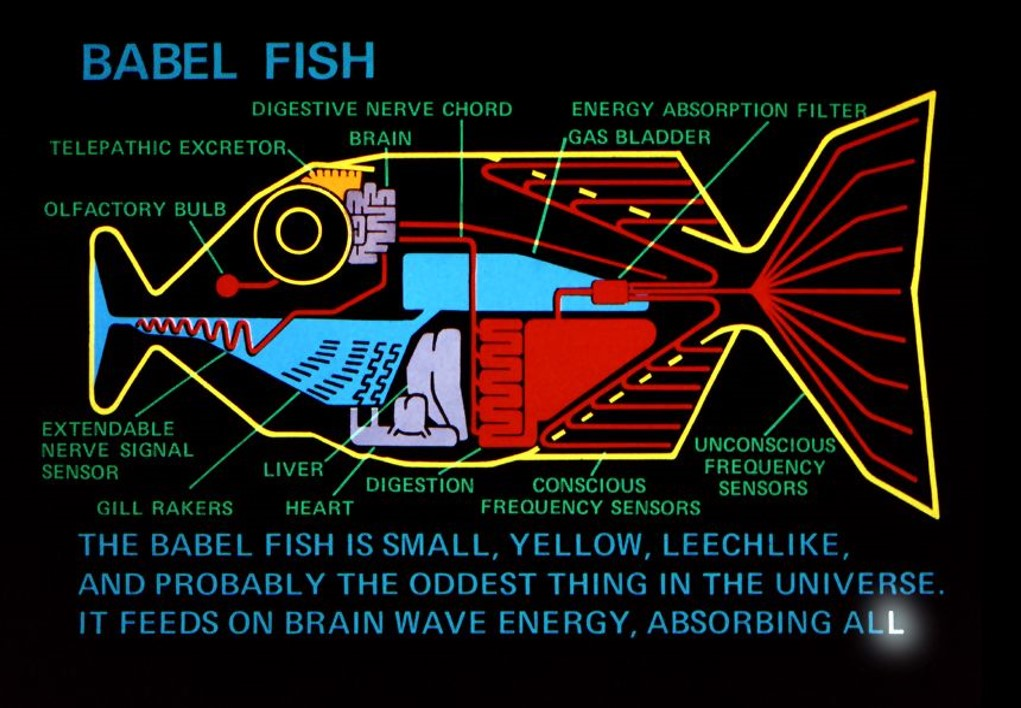# Type provider patterns

Providers for a specific data source

 1: 2:  let wb = WorldBankData.GetDataContext() wb.Countries.India.Indicators.Population, total 

Parameterized provider for a data format

 1: 2:  type Rss = XmlProvider<"data/bbc.xml"> Rss.Load(url).Channel.Description 

# The Star Wars social network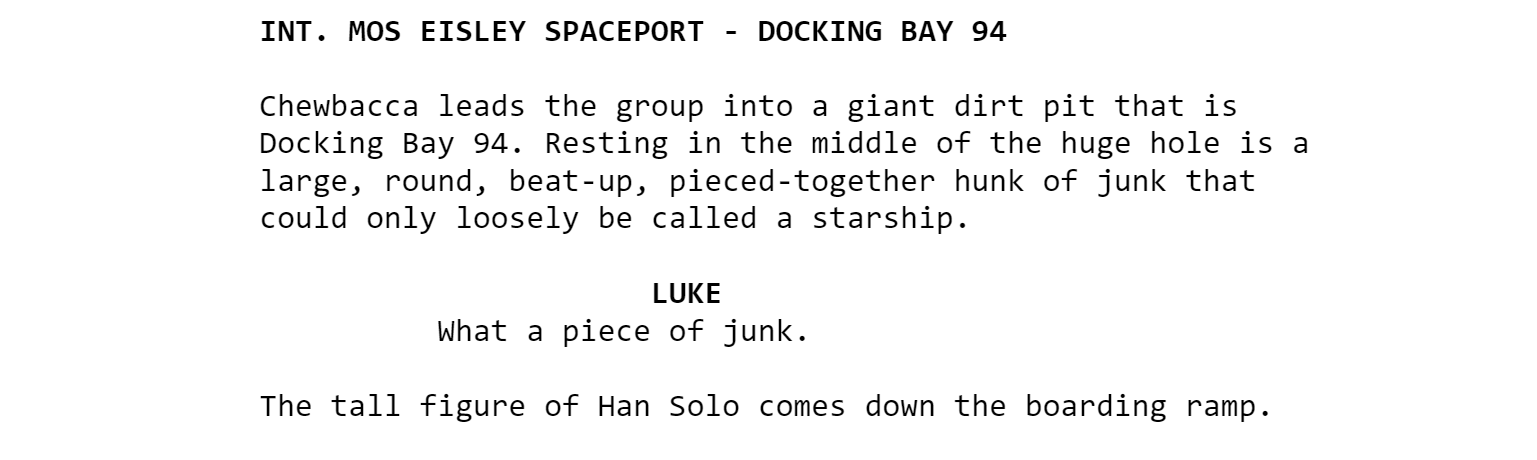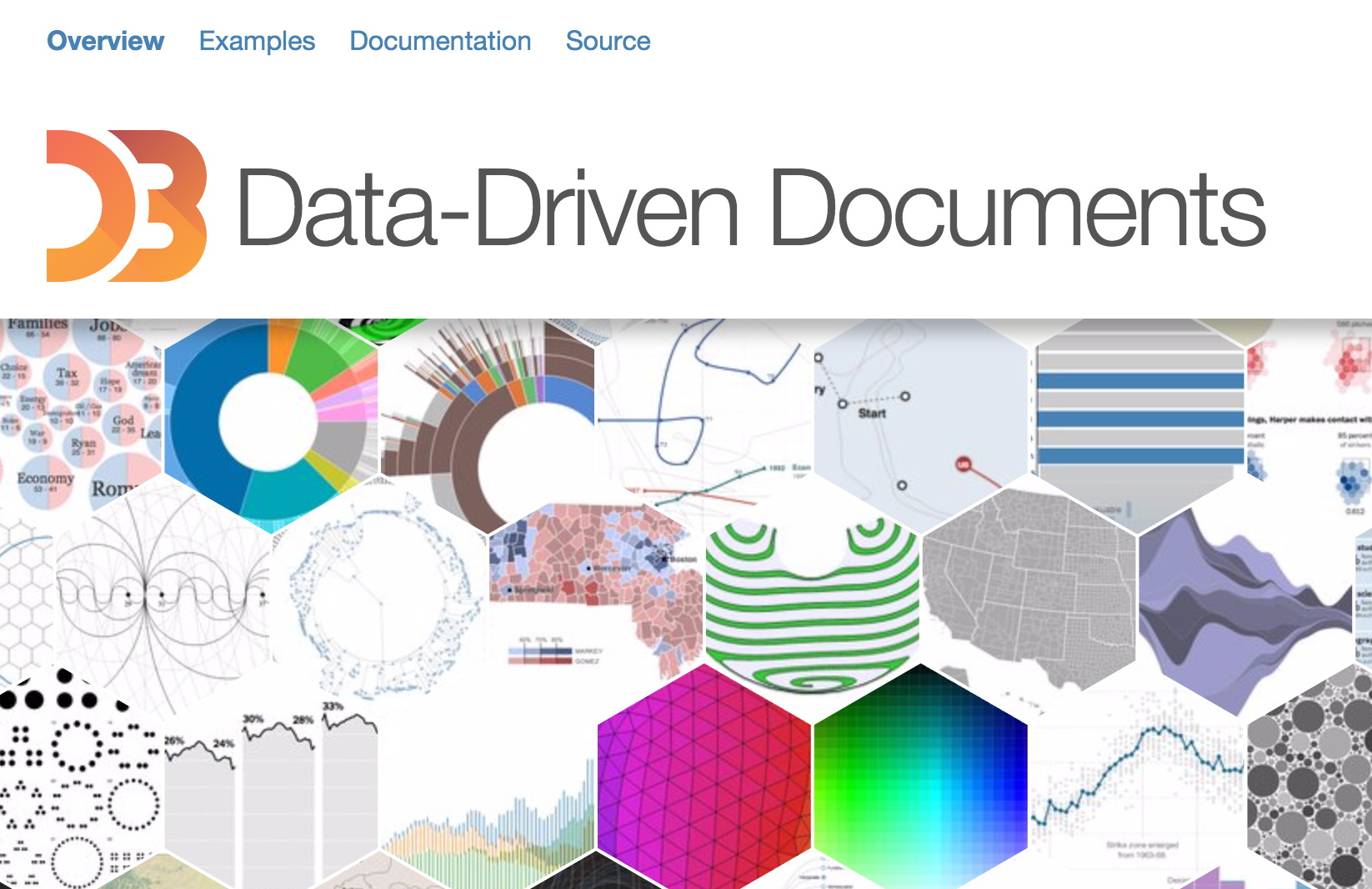# D3.js visualizations

Gallery of examples

# D3.js social network visualization

Force-directed network layout

# Social network analysis

• Who is the most central character?
• How to the movies compare between themselves?

# The R language• "domain-specific" language for statistical analysis

# Very quick R intro

 1: 2: 3: 4: 5: 6: 7: 8:  # assignment x <- 1 x = 1 # variable and function names x x.y read.csv 

# Very quick R intro: pipeline

## |> turns into %>%

 1: 2: 3: 4: 5:  install.packages("magrittr") library(magrittr) xs <- c(1,2,3,4,5,6,7,8,9,10) xs %>% mean 

# Network analysis with igraph

 1: 2:  install.packages("igraph") library(igraph) 

# Creating igraph network

 1: 2: 3:  library(igraph) g <- graph(edges) 
• edges = list of nodes

n1, n2, n3, n4, n5, ...
represents (n1, n2), (n3, n4), ...

# Calculating degree

 1:   d <- degree(graph) 

# F#

 1: 2: 3:  open RProvider.igraph let degree = R.degree(network) 

# F#

export JSON into list of edges

# R

perform the network analysis

# Degree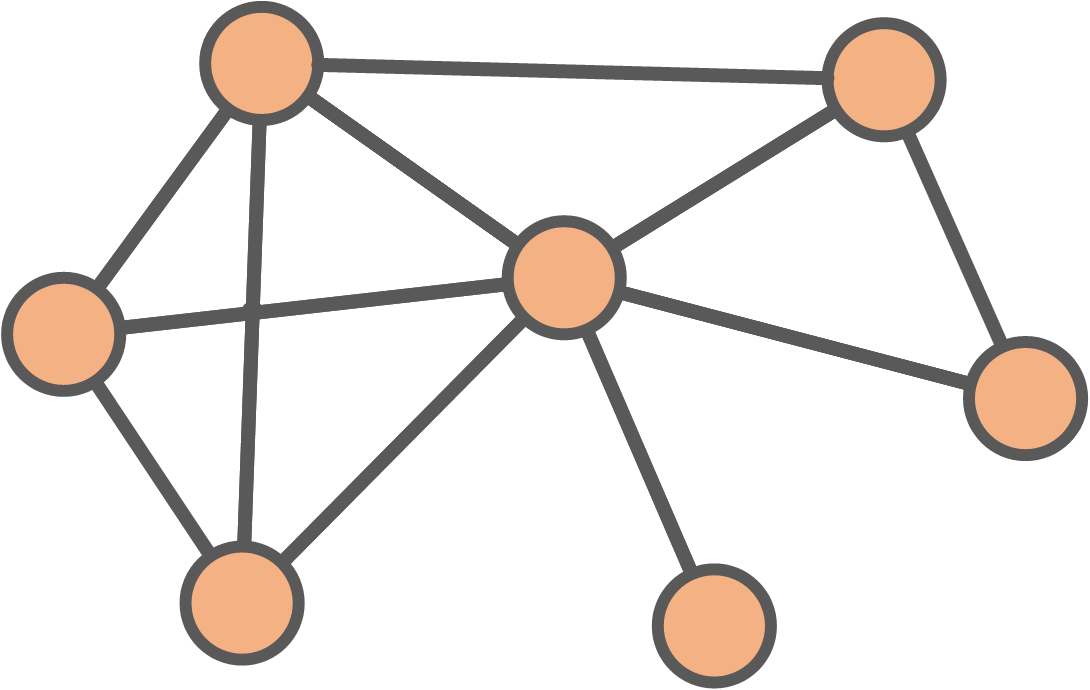# Degree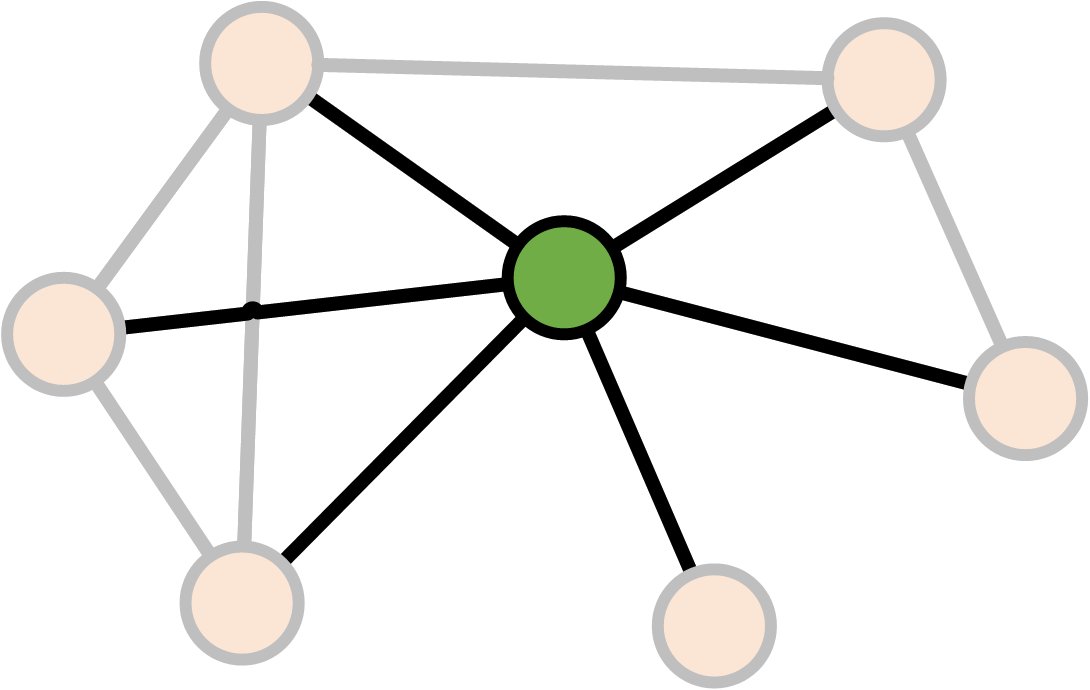# Degree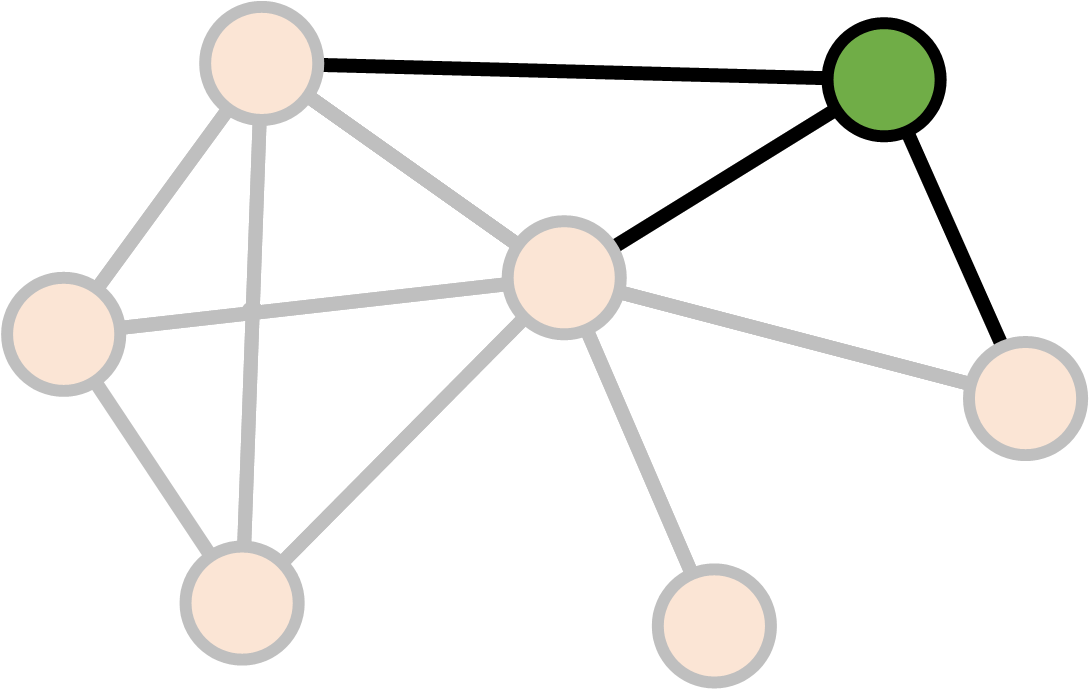# Degree

$\text{Degree}(v) = \text{Number of links }v \leftrightarrow v' \\ v \neq v'$

# Betweenness# Betweenness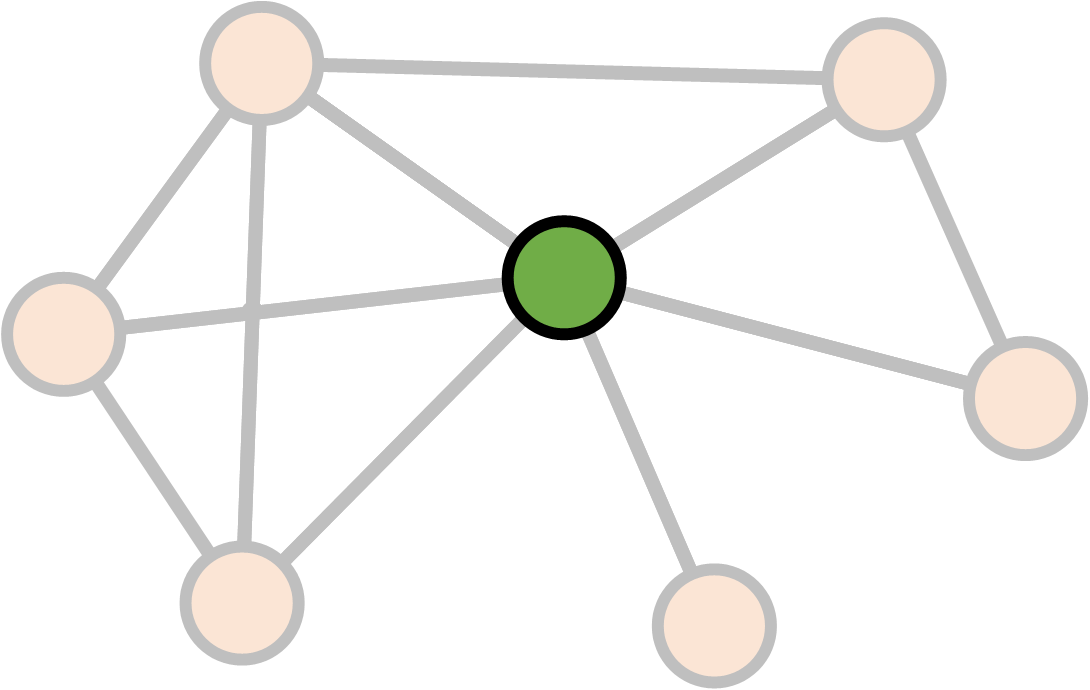# Betweenness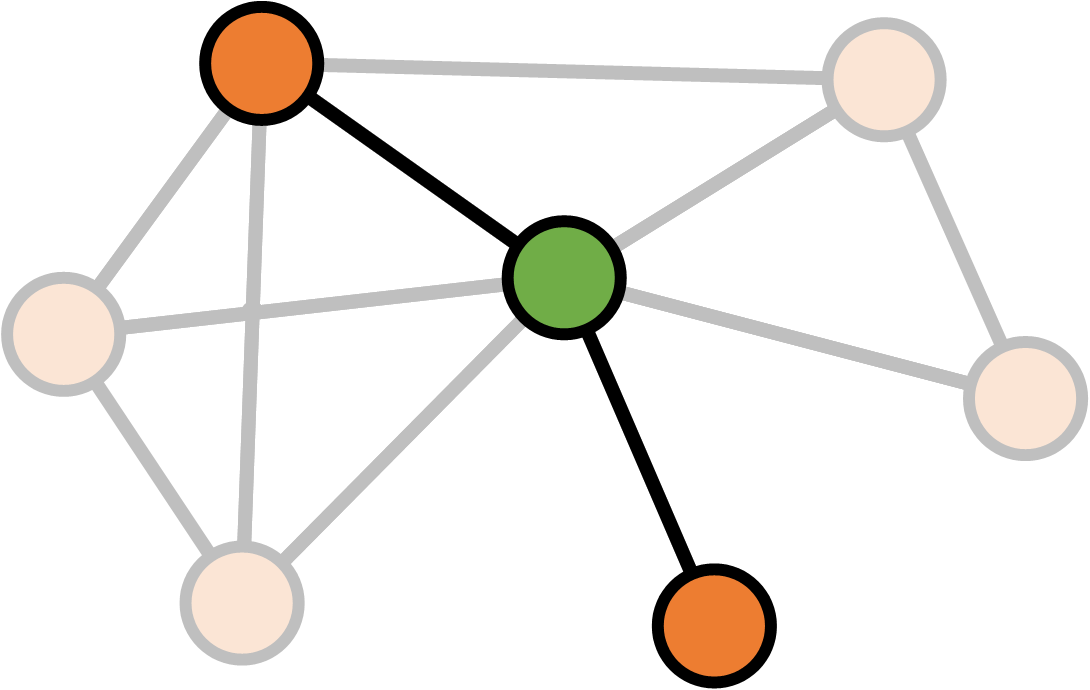# Betweenness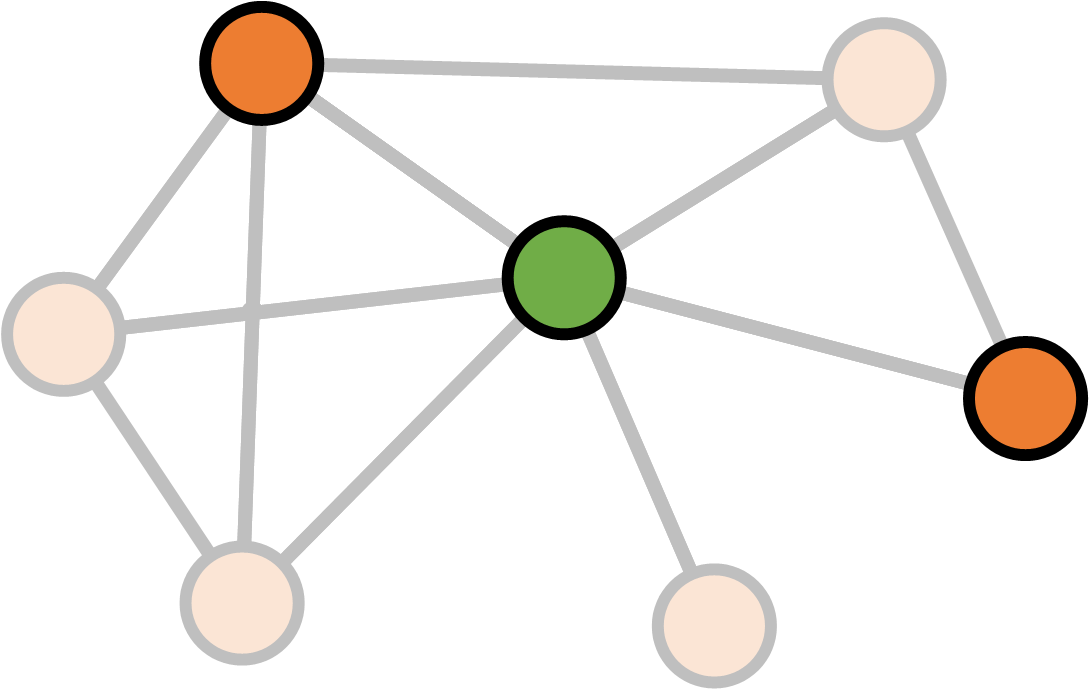# Betweenness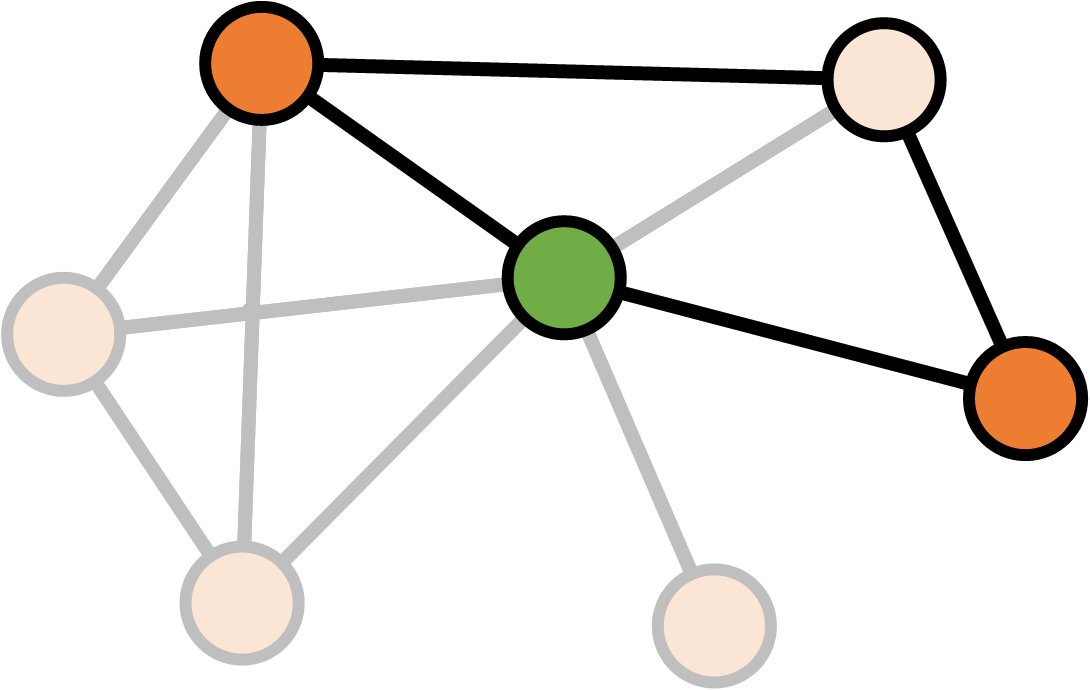# Betweenness

$S_v = \text{Number of shortest paths between a and b through v} \\ S = \text{Number of shortest paths between a and b} \\ \\ \text{Betweenness}(v)_{ab} = \frac{S_v}{S}$

# Betweenness

$S_v = \text{Number of shortest paths between a and b through v} \\ S = \text{Number of shortest paths between a and b} \\ \\ \text{Betweenness}(v) = \sum_{ab} \frac{S_v}{S}$

# Network structure

How do the the movies differ?

• Size
• Density
• Clustering coefficient

# Density# Density# Density

\begin{align} \text{Density} &= \frac{\text{Existing connections}}{\text{Potential connections}} \\ & \\ &= \frac{\text{Existing connections}}{\frac{1}{2}N(N-1)} \end{align}

# Clustering coefficient# Clustering coefficient# Clustering coefficient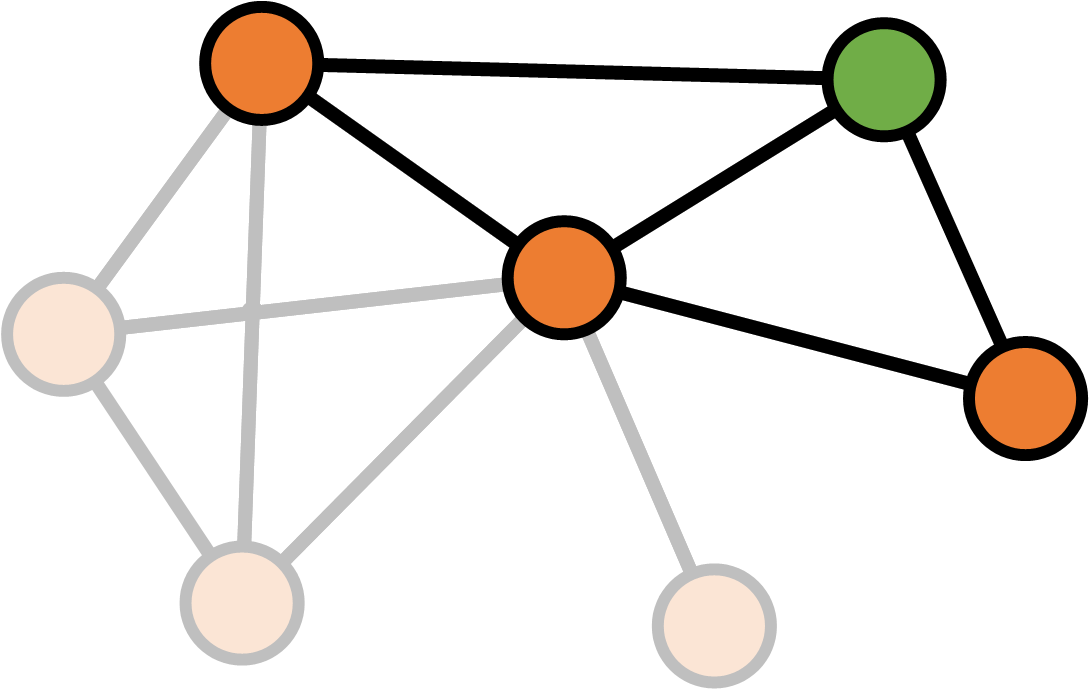# Clustering coefficient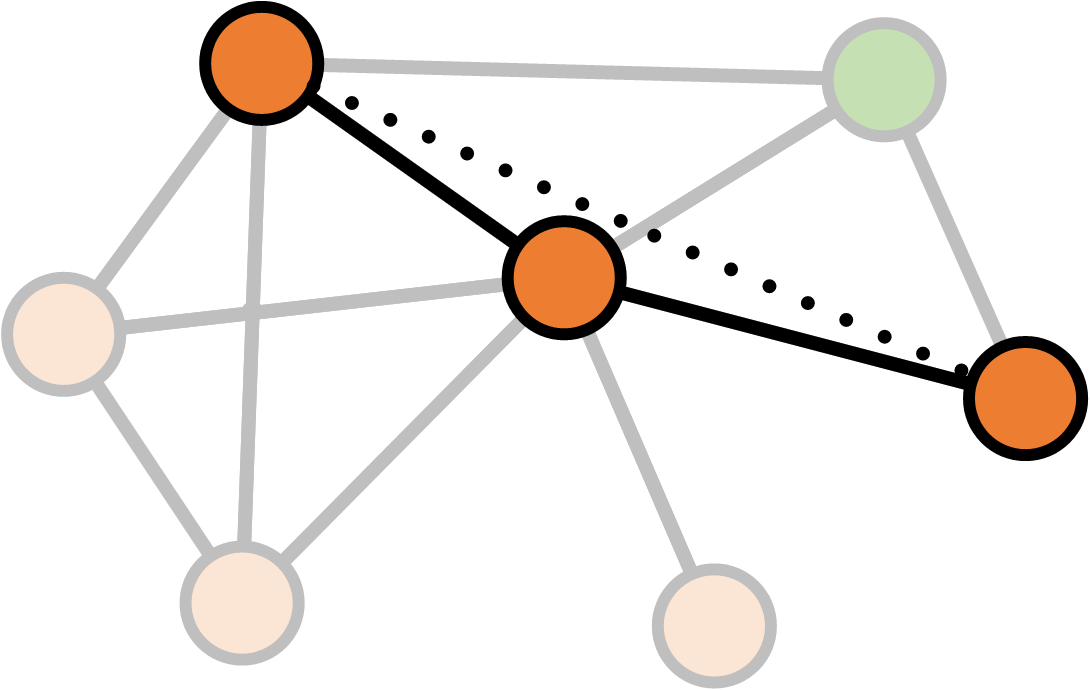# Clustering coefficient# Clustering coefficient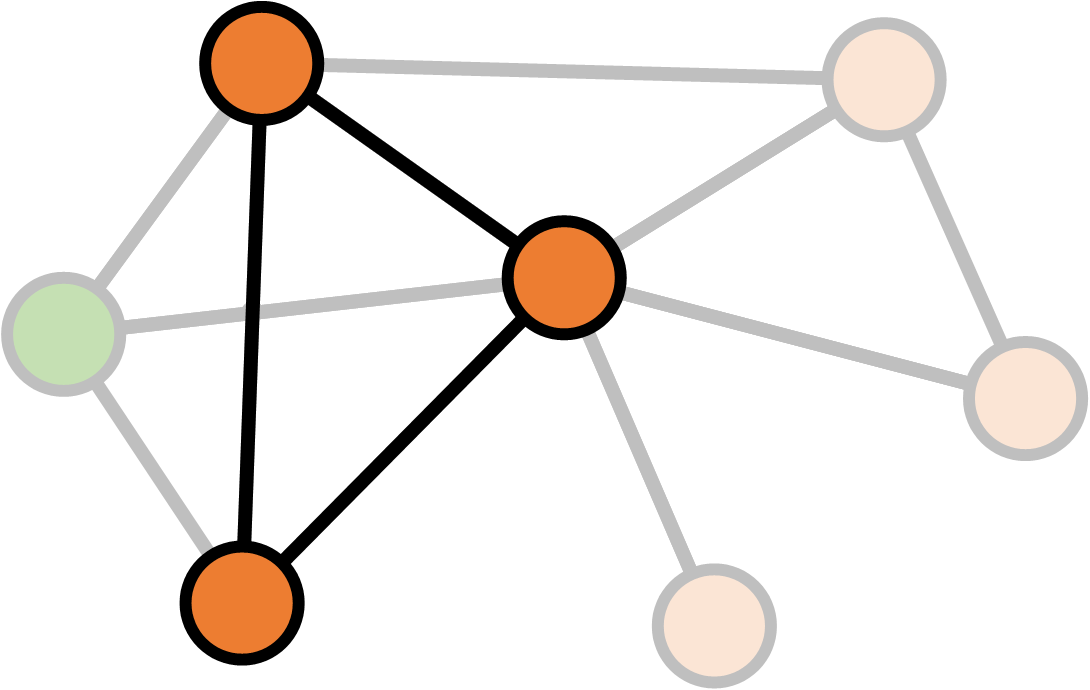# Clustering coefficient

$K_v = \text{Number of neighbours of v} \\ E_v = \text{Number of links between neighbours of v} \\ \\ \text{Clustering}(v) = \frac{E_v}{\frac{1}{2} K_v (K_v - 1)}$

# Clustering coefficient

$K_v = \text{Number of neighbours of v} \\ E_v = \text{Number of links between neighbours of v} \\ \\ \text{Clustering}(\text{network}) = \frac{1}{N} \sum_v \frac{E_v}{\frac{1}{2} K_v (K_v - 1)}$

# CONCLUSIONS

non-profit books and tutorials

cross-platform community data science

## F# Software Foundation

commercial support open-source contributions

machine learning www.fsharp.org web and cloud

consulting user groups research

# The Learning Pyramid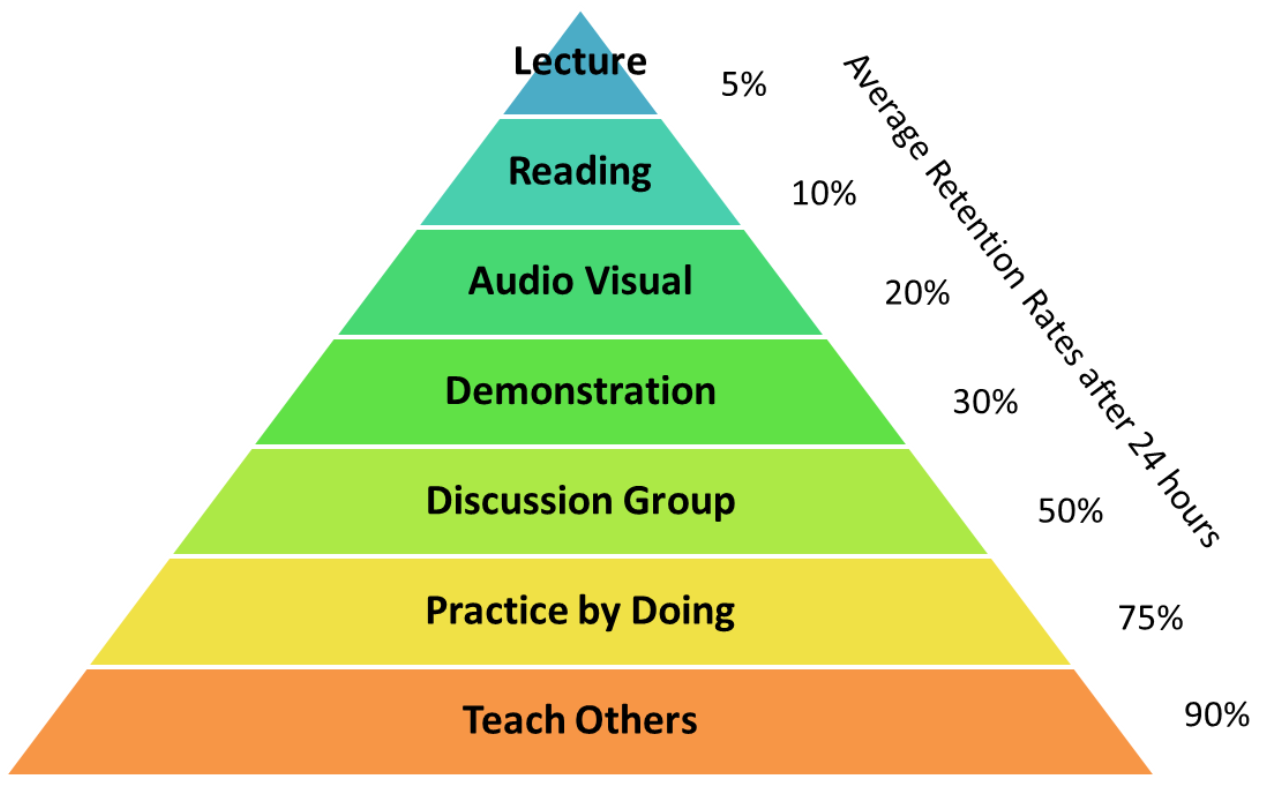## Community chat and Q&A

• StackOverflow F# tag

# F# Books and Resources